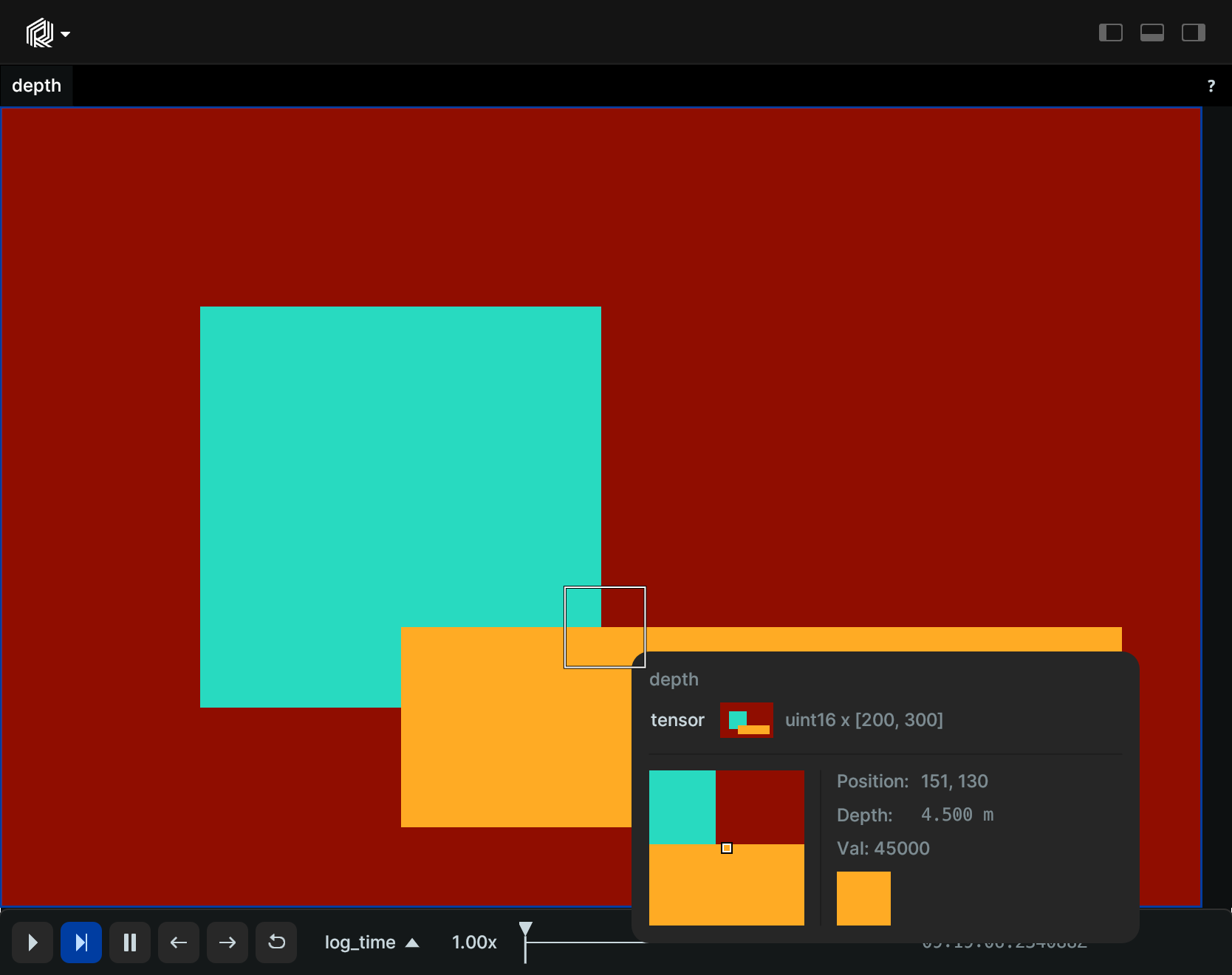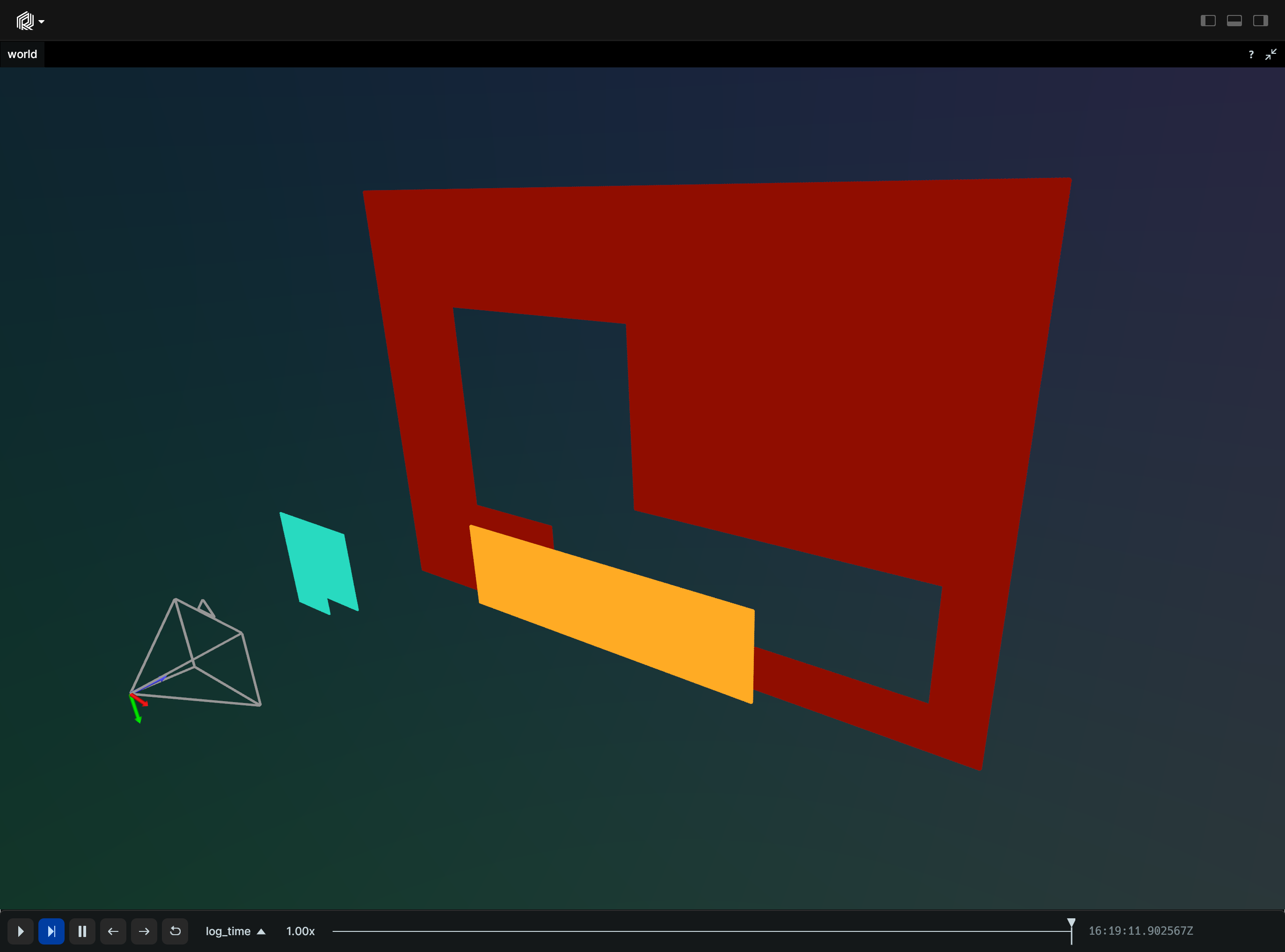# DepthImage

A depth image is a 2D image containing depth information. It is a 2D tensor with a single channel of type `uint16`, `float32`, or `float64`. It can be displayed in a 3D viewer when combined with a pinhole camera.

## Components and APIs

Primary component: `tensor`

Secondary components: `draw_order`

Python APIs: log_depth_image,

Rust API: Tensor

## Simple example

``````"""Create and log a depth image."""

import numpy as np
import rerun as rr

# Create a dummy depth image
image = 65535 * np.ones((200, 300), dtype=np.uint16)
image[50:150, 50:150] = 20000
image[130:180, 100:280] = 45000

rr.init("rerun_example_depth_image", spawn=True)

# Log the tensor, assigning names to each dimension
rr.log_depth_image("depth", image, meter=10000.0)
``````## Depth to 3D example

``````"""Create and log a depth image and pinhole camera."""

import numpy as np
import rerun as rr

# Create a dummy depth image
image = 65535 * np.ones((200, 300), dtype=np.uint16)
image[50:150, 50:150] = 20000
image[130:180, 100:280] = 45000

rr.init("rerun_example_depth_image", spawn=True)

# If we log a pinhole camera model, the depth gets automatically back-projected to 3D
rr.log_pinhole(
"world/camera",
width=image.shape,
height=image.shape,
focal_length_px=200,
)

# Log the tensor, assigning names to each dimension
rr.log_depth_image("world/camera/depth", image, meter=10000.0)
``````